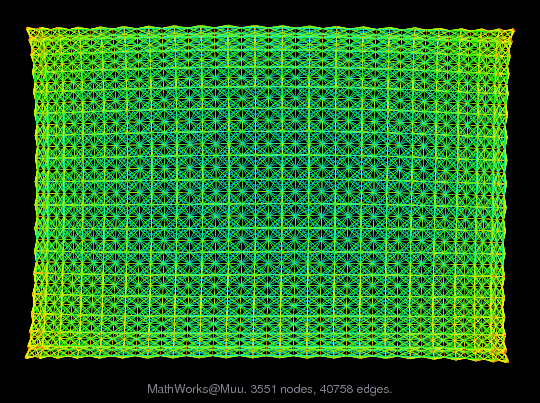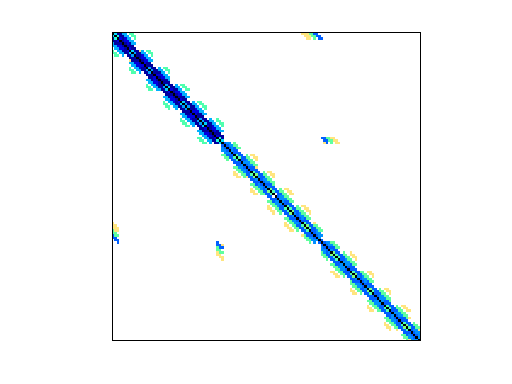Matrix: MathWorks/Muu

Description: The MathWorks, Inc: symmetric positive definite matrix(undirected graph drawing)• Matrix group: MathWorks
• download as a MATLAB mat-file, file size: 336 KB. Use UFget(1331) or UFget('MathWorks/Muu') in MATLAB.

 Matrix properties number of rows 7,102 number of columns 7,102 nonzeros 170,134 structural full rank? yes structural rank 7,102 # of blocks from dmperm 2 # strongly connected comp. 2 explicit zero entries 0 nonzero pattern symmetry symmetric numeric value symmetry symmetric type real structure symmetric Cholesky candidate? yes positive definite? yes

 author B. Cheng editor T. Davis date 2006 kind structural problem 2D/3D problem? yes

 Ordering statistics: result nnz(chol(P*(A+A'+s*I)*P')) with AMD 187,090 Cholesky flop count 7.5e+06 nnz(L+U), no partial pivoting, with AMD 367,078 nnz(V) for QR, upper bound nnz(L) for LU, with COLAMD 402,408 nnz(R) for QR, upper bound nnz(U) for LU, with COLAMD 1,471,728

 SVD-based statistics: norm(A) 0.000839895 min(svd(A)) 1.09738e-05 cond(A) 76.5362 rank(A) 7,102 sprank(A)-rank(A) 0 null space dimension 0 full numerical rank? yes

 singular values (MAT file): click here SVD method used: s = svd (full (A)) ; status: ok